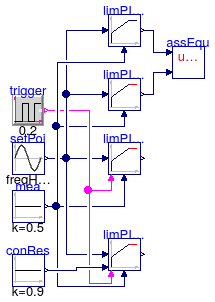## Buildings.Controls.Continuous.Validation

Collection of validation models

### Information

This package contains validation models for the classes in Buildings.Controls.Continuous.

Note that most validation models contain simple input data which may not be realistic, but for which the correct output can be obtained through an analytic solution. The examples plot various outputs, which have been verified against these solutions. These model outputs are stored as reference data and used for continuous validation whenever models in the library change.

Extends from Modelica.Icons.ExamplesPackage (Icon for packages containing runnable examples).

### Package Content

Name DescriptionLimPIDReset Test model for PID controller with optional intgerator resetOffTimerNonZeroStart Test model for off timer with negative start time

##Buildings.Controls.Continuous.Validation.LimPIDReset

Test model for PID controller with optional intgerator reset### Information

This model tests the implementation the Buildings.Controls.Continuous.LimPID with integrator reset.

The instance `limPIOri` is the original implementation of the controller from the Modelica Standard Library. The instance `limPIWithReset` is the implementation from this library with integrator reset enabled. Whenever the boolean pulse input becomes true, the integrator is reset to `y_reset`.

Extends from Modelica.Icons.Example (Icon for runnable examples).

### Modelica definition

model LimPIDReset "Test model for PID controller with optional intgerator reset" extends Modelica.Icons.Example; Modelica.Blocks.Sources.Sine setPoi(freqHz=1) "Set point signal"; Buildings.Controls.Continuous.LimPID limPIDPar( yMax=1, yMin=-1, reset=Buildings.Types.Reset.Parameter, y_reset=0.2, Ti=20, Td=10, k=0.2, controllerType=Modelica.Blocks.Types.SimpleController.PID, initType=Modelica.Blocks.Types.InitPID.InitialOutput, y_start=0.3) "PId controller with integrator reset to a parameter value"; Modelica.Blocks.Sources.Constant mea(k=0.5) "Measured signal"; Buildings.Controls.Continuous.LimPID limPIDDef( initType=Modelica.Blocks.Types.InitPID.InitialState, Td=10, k=1, Ti=1, yMax=100, yMin=-100, controllerType=Modelica.Blocks.Types.SimpleController.PID, xi_start=0.2, xd_start=0.1) "PID controller without integrator reset"; Modelica.Blocks.Sources.BooleanPulse trigger( width=50, startTime=0.1, period=0.2) "Boolean pulse to reset integrator"; Buildings.Controls.Continuous.LimPID limPIDInp( y_reset=0.2, Td=10, reset=Buildings.Types.Reset.Input, yMax=1, yMin=-1, k=0.2, Ti=20, controllerType=Modelica.Blocks.Types.SimpleController.PID, initType=Modelica.Blocks.Types.InitPID.InitialOutput, y_start=0.3) "PId controller with integrator reset to an input value"; Modelica.Blocks.Sources.Constant conRes(k=0.9) "Signal to which integrator will be reset to"; Modelica.Blocks.Continuous.LimPID limPIDOri( initType=Modelica.Blocks.Types.InitPID.InitialState, Td=10, k=1, Ti=1, yMax=100, yMin=-100, controllerType=Modelica.Blocks.Types.SimpleController.PID, xi_start=0.2, xd_start=0.1) "PID controller from Standard Modelica Library"; Buildings.Utilities.Diagnostics.AssertEquality assEqu; equation connect(setPoi.y, limPIDPar.u_s); connect(mea.y, limPIDPar.u_m); connect(setPoi.y, limPIDDef.u_s); connect(mea.y, limPIDDef.u_m); connect(trigger.y, limPIDPar.trigger); connect(setPoi.y, limPIDInp.u_s); connect(mea.y, limPIDInp.u_m); connect(trigger.y, limPIDInp.trigger); connect(conRes.y, limPIDInp.y_reset_in); connect(setPoi.y, limPIDOri.u_s); connect(mea.y, limPIDOri.u_m); connect(assEqu.u1, limPIDOri.y); connect(limPIDDef.y, assEqu.u2); end LimPIDReset;

##Buildings.Controls.Continuous.Validation.OffTimerNonZeroStart

Test model for off timer with negative start time### Information

This model tests the implementation the Buildings.Controls.Continuous.OffTimer for negative start time.

Extends from Buildings.Controls.Continuous.Examples.OffTimer (Example model for off timer).

### Modelica definition

model OffTimerNonZeroStart "Test model for off timer with negative start time" extends Buildings.Controls.Continuous.Examples.OffTimer(booleanPulse( startTime=-1)); end OffTimerNonZeroStart;